# Ounce

749 calories in a 7 - ounce serving . What is the unit rate?

u =  107 cal/oz

### Step-by-step explanation:Did you find an error or inaccuracy? Feel free to write us. Thank you!

Showing 1 comment:Alex
107 cal/ozTips to related online calculators
Check out our ratio calculator.

## Related math problems and questions:

• Calories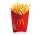A large order of McDonald's french fries has 500 calories. Of this total, 220 calories are from fat. Find the ratio of the calories from fat to total calories in a large order of McDonald's french fries.
• Unit rateFind unit rate: 6,840 customers in 45 days
• Ratio6 numbers are in the ratio 1:5:1:5:5:5. Their sum is 242. What are the numbers?
• RemaindersIt is given a set of numbers { 170; 244; 299; 333; 351; 391; 423; 644 }. Divide this numbers by number 66 and determine set of remainders. As result write sum of this remainders.
• Food weightStacie is a resident at the medical facility where you work. You are asked to chart the amount of solid food that she consumes. For the noon meal, today she ate 1/2 of a 3-ounce serving of meatloaf, 3/4 of her 3-ounce serving of mashed potatoes, and 1/3 o
• Ornamental shrubsChildren committed to planting 240 ornamental shrubs. Their commitment, however, exceeded by 48 shrubs. Write the ratio of actually planted shrubs and commitment by lowest possible integers a/b.
• Kilowatt-hours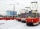If the Lewis family used 648 kilowatt-hours of electricity in 12 days, how many kilowatt-hours should they use in 24 days at the same usage rate?
• Moneys in triple ratioMilan, John and Lili have a total 344 euros. Their amounts are in the ratio 1:2:5. Determine how much each of them has?
• How manyHow many integers are greater than 547/3 and less than 931/4?
• RatiosReduce the numbers: 50 in a 1:2 ratio 111 at a ratio of 2:3 70 at 10:50 560 at a ratio of 3:8
• Potatoes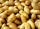Could 446 tons of potatoes (ρ = 771 kg/m³) fit in a warehouse with a volume of 699 m³?
• Kate shares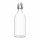Kate shares a 64-ounce bottle of apple cider with five friends. Each person's serving will be the same number of ounces. Between what two whole number of ounces will each person's serving to be? Explain using division.
• SymbolsIf 2*3 = 60 ; 3*4 = 120 and 4*5 = 200, what is 2*5?
• Above 100%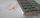Find 340 percent of the numbers 45 and 55.
• BuildingAt the building, we divided 240 boards into two piles in a 5: 3 ratio. How many were fewer boards in the lower pile?
• ŽSRCalculate fixed annual personnel costs of operating monorail line 118 km long if every 5 km is a station, which serves three people - one dispatcher and two switchmen in 4-shift operation. Consider the average salary of the employee 885 €.
• ConcertOn a Concert were sold 150 tickets for CZK 360, 235 tickets for 240 CZK and 412 for 180 CZK. How much was the total revenues for tickets?